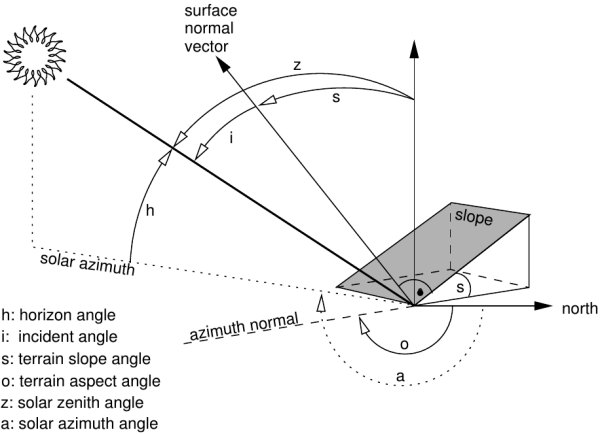Note: This document is for an older version of GRASS GIS that will be discontinued soon. You should upgrade, and read the current manual page.

## NAME

i.topo.corr - Computes topographic correction of reflectance.

## KEYWORDS

imagery, terrain, topographic correction

## SYNOPSIS

i.topo.corr
i.topo.corr --help
i.topo.corr [-is] [input=name[,name,...]] output=name basemap=name zenith=float [azimuth=float] [method=string] [--overwrite] [--help] [--verbose] [--quiet] [--ui]

### Flags:

-i
Output sun illumination terrain model
-s
Scale output to input and copy color rules
--overwrite
Allow output files to overwrite existing files
--help
Print usage summary
--verbose
Verbose module output
--quiet
Quiet module output
--ui
Force launching GUI dialog

### Parameters:

input=name[,name,...]
Name of reflectance raster maps to be corrected topographically
output=name [required]
Name (flag -i) or prefix for output raster maps
basemap=name [required]
Name of input base raster map (elevation or illumination)
zenith=float [required]
Solar zenith in degrees
azimuth=float
Solar azimuth in degrees (only if flag -i)
method=string
Topographic correction method
Options: cosine, minnaert, c-factor, percent
Default: c-factor

## DESCRIPTION

i.topo.corr is used to topographically correct reflectance from imagery files, e.g. obtained with i.landsat.toar, using a sun illumination terrain model. This illumination model represents the cosine of the incident angle i, i.e. the angle between the normal to the ground and the sun rays.

Note: If needed, the sun position can be calculated for a given date with r.sunmask.Figure showing terrain and solar angles

Using the -i flag and given an elevation basemap (metric), i.topo.corr creates a simple illumination model using the formula:

• cos_i = cos(s) * cos(z) + sin(s) * sin(z) * cos(a - o)
where, i is the incident angle to be calculated, s is the terrain slope angle, z is the solar zenith angle, a the solar azimuth angle, o the terrain aspect angle.

For each band file, the corrected reflectance (ref_c) is calculate from the original reflectance (ref_o) using one of the four offered methods (one lambertian and two non-lambertian).

### Method: cosine

• ref_c = ref_o * cos_z / cos_i

### Method: minnaert

• ref_c = ref_o * (cos_z / cos_i) ^k
where, k is obtained by linear regression of
ln(ref_o) = ln(ref_c) - k ln(cos_i/cos_z)

### Method: c-factor

• ref_c = ref_o * (cos_z + c)/ (cos_i + c)
where, c is a/m from ref_o = a + m * cos_i

### Method: percent

We can use cos_i to estimate the percent of solar incidence on the surface, then the transformation (cos_i + 1)/2 varied from 0 (surface in the side in opposition to the sun: infinite correction) to 1 (direct exhibition to the sun: no correction) and the corrected reflectance can be calculated as
• ref_c = ref_o * 2 / (cos_i + 1)

## NOTES

1. The illumination model (cos_i) with flag -i uses the actual region as limits and the resolution of the elevation map.
2. The topographic correction use the full reflectance file (null remain null) and its resolution.
3. The elevation map to calculate the illumination model should be metric.

## EXAMPLES

First, make a illumination model from the elevation map (here, SRTM). Then make perform the topographic correction of e.g. the bands toar.5, toar.4 and toar.3 with output as tcor.toar.5, tcor.toar.4, and tcor.toar.3 using c-factor (= c-correction) method:

```# first pass: create illumination model
i.topo.corr -i base=SRTM zenith=33.3631 azimuth=59.8897 output=SRTM.illumination

# second pass: apply illumination model
i.topo.corr base=SRTM.illumination input=toar.5,toar.4,toar.3 output=tcor \
zenith=33.3631 method=c-factor
```

## REFERENCES

• Law K.H. and Nichol J, 2004. Topographic Correction For Differential Illumination Effects On Ikonos Satellite Imagery. International Archives of Photogrammetry Remote Sensing and Spatial Information, pp. 641-646.
• Meyer, P. and Itten, K.I. and Kellenberger, KJ and Sandmeier, S. and Sandmeier, R., 1993. Radiometric corrections of topographically induced effects on Landsat TM data in alpine terrain. Photogrammetric Engineering and Remote Sensing 48(17).
• Riaño, D. and Chuvieco, E. and Salas, J. and Aguado, I., 2003. Assessment of Different Topographic Corrections in Landsat-TM Data for Mapping Vegetation Types. IEEE Transactions On Geoscience And Remote Sensing, Vol. 41, No. 5
• Twele A. and Erasmi S, 2005. Evaluating topographic correction algorithms for improved land cover discrimination in mountainous areas of Central Sulawesi. Göttinger Geographische Abhandlungen, vol. 113.

## AUTHOR

Dept. Biodiversity and Environmental Management, University of León, Spain

Figure derived from Neteler & Mitasova, 2008.

## SOURCE CODE

Available at: i.topo.corr source code (history)

Latest change: Mon Nov 18 20:15:32 2019 in commit: 1a1d107e4f6e1b846f9841c2c6fabf015c5f720d

Note: This document is for an older version of GRASS GIS that will be discontinued soon. You should upgrade, and read the current manual page.

© 2003-2023 GRASS Development Team, GRASS GIS 7.8.8dev Reference Manual